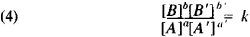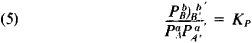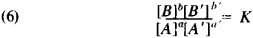# law of mass action

(redirected from C. Guldberg)
Also found in: Dictionary, Thesaurus, Medical.

## law of mass action

[′lȯ əv ¦mas ′ak·shən]
(chemistry)
The law stating that the rate at which a chemical reaction proceeds is directly proportional to the molecular concentrations of the reacting compounds.
McGraw-Hill Dictionary of Scientific & Technical Terms, 6E, Copyright © 2003 by The McGraw-Hill Companies, Inc.
The following article is from The Great Soviet Encyclopedia (1979). It might be outdated or ideologically biased.

## Mass Action, Law of

one of the fundamental laws of physical chemistry; it establishes the dependence of the rate of a chemical reaction on the concentrations of the reacting substances and the relationship between the concentrations (or activities) of the products of the reaction and the original substances in the state of chemical equilibrium. The Norwegian scientists C. Guldberg and P. Waage, who formulated the law of mass action in 1864-67, called the amount of the substance per unit volume, that is, its concentration, the “active mass” of the substance, hence the name of the law.

If in an ideal gas mixture or an ideal liquid solution the reaction

(1) aA + a′ A′ = bB +b′B′

(A, A, … are substances and a, a′, … are the stoichiometric coefficients) takes place, then according to the law of mass action, the reaction rate in the forward direction is

(2) r+ = k+[A]a[A′]a′

Here [A ] is the concentration of substances A, . . , and k+ is the reaction constant in the forward direction. k+ depends on the temperature and, in a liquid solution, also on the pressure; the latter dependence is significant only at high pressures. The form of equation (2) is determined by the fact that a necessary condition for the elementary reaction event is the collision of the molecules of the original substances, namely their encounter in a certain small volume (on the order of the size of the molecules). The probability of finding a molecule of the type A at a given moment in a given small volume is proportional to [A ]; the probability of simultaneously finding in this volume a molecules of A and a′ molecules of A′ is, according to the theorem on the probability of a complex event, proportional to [A ]a[A′ ]a′. The number of collisions of the molecules of the original substances per unit volume per unit time is proportional to this quantity. A certain portion of these collisions leads to reaction. These considerations lead to equation (2). Monomolecular reactions require a separate analysis.

The rate of reaction (1) in the reverse direction is

(3) r- = k-[B]b[B′]b′

If the reaction is reversible, that is, if it proceeds simultaneously in opposite directions, then the observed reaction rate r = r+ - r-. At r+ = r-, chemical equilibrium is achieved. Then, according to equations (2) and (3),where K = k+/k- is the equilibrium constant.

For gas reactions the equivalent equilibrium expressionis customarily used, where PA is the partial pressure of substances A. …

Equations (2) and (3) are applicable to a simple one-step reaction and to the separate steps of a complex reaction but not to a complex reaction as a whole. Equations (4) and (5), representing the law of mass action for the equilibrium case, are also valid in the case of a complex reaction.

The general condition for equilibrium with respect to reaction (1), the applicability of which is not limited to ideal systems, is the equationin which [A ] is the activity of substance A … Equation (6) is derived from thermodynamic principles. The law of mass action for the equilibrium case is used to calculate the maximum attainable degrees of conversion in reversible reactions. The latter include important industrial processes, such as ammonia synthesis, sulfur dioxide oxidation, and many other reactions. Based on reaction rates, the law of mass action is used to obtain kinetic equations applicable in the design of chemical equipment.

M. I. TEMKIN